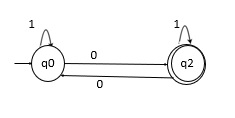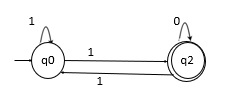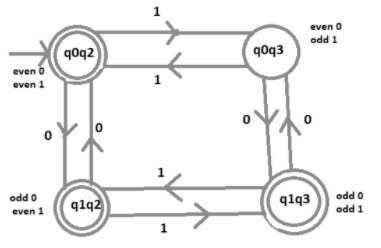# Design a DFA machine accepting odd numbers of 0’s or even numbers of 1’s

A Deterministic Finite automaton (DFA) is a five tuples

M=(Q, Σ, δ,q0,F)

Where,

• Q : Finite set called states.
• Σ : Finite set called alphabets.
• δ : Q × Σ → Q is the transition function.
• q0 ϵ Q is the start or initial state.
• F : Final or accept state.

## Problem

Construct a DFA machine accepting odd numbers of 0’s or even numbers of 1’s.

## Solution

Design two separate machines for the two conditions over an alphabet Σ={0,1} −

• DFA accepting only odd number of 0’s

• DFA accepting only even number of 1’s

DFA accepting only odd number of 1’s over an alphabet Σ={0,1}

The language L= {1,10,1110,1011,10101,100101,….}DFA accepting only even number of 1’s over an alphabet Σ={0,1}

The language L= {11,101,11110,10111,101101,1001011,….}Now merge two transition diagrams to generate a machine accepting odd number of 0’s or even number of 1’s